# Overview

WorldView-1, WorldView-2 and WorldView-3 are commercial earth observation satellites. Details about the sensors are provided at Digital Globe's collection of Spacecraft Data Sheets.

It is recommended -- actually it is a necessity! -- to have a look at the documentation explaining Digital Globe's products metadata. Note, all of DigitalGlobe’s satellites collect data using an 11 bit dynamic range. For technical reasons, products are delivered as either 16-bit or 8-bit data.

## Availability (Sample Data)

• Search for commercial satellite image providers in the internet.

## Modules overview

### Outside of GRASS

Satellite imagery can be managed and, at some extent, pre-processed with various GDAL utilities.

### Inside GRASS GIS

GRASS GIS features a complete set of modules and various add-ons enabling pre- and post-processing of WorldView satellite imagery. The following lists offer an overview of related modules and add-ons.

## Pre-Processing

### Overview

Typically, multispectral satellite data are converted into physical quantities such as Radiance or Reflectance before they are subjected in multispectral analysis techniques (image interpretation, band arithmetic, vegetation indices, matrix transformations, etc.). The latter can be differentiated in Top of Atmosphere Reflectance (ToAR) which does not account for atmospheric effects (absorption or scattering) and in Top of Canopy Reflectance (ToCR) which introduces a "correction" for atmospheric effects.

In order to derive Reflectance values, likewise as with remotely sensed data acquired by other sensors, WorldView raw image digital numbers (DNs) need to be converted to at-sensor spectral Radiance values. At-sensor spectral Radiance values are an important input for the equation to derive Reflectance values. Note, Spectal Radiance is the measure of the quantity of radiation that hits the sensor and typically expressed in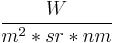$\frac{W}{m^2*sr*nm}$, that is watts per unit source area, per unit solid angle, and per unit wavelength.$L_{\lambda\text{Pixel, Band}} = \frac{K_{\text{Band}} * q_{\text{Pixel, Band}}}{\Delta\lambda_{\text{Band}}}$
where:
•$L_{\lambda\text{Pixel,Band}}$: Top-of-Atmosphere Spectral Radiance image pixels [W-m-2-sr-1-μm-1]
•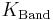$K_{\text{Band}}$: the absolute radiometric calibration factor [W-m-2-sr-1-count-1] for a given band
•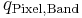$q_{\text{Pixel,Band}}$: radiometrically corrected image pixels [counts or Digital Numbers]
•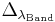$\Delta_{\lambda_{\text{Band}}}$: the effective bandwidth [μm] for a given band

• Converting to Top of Atmosphere Reflectance, also referred to as Planetary Reflectance, can be done by using the following equation:$\rho_p = \frac{\pi * L\lambda * d^2}{ESUN\lambda * cos(\Theta_S)}$

where:

•$\rho$ - Unitless Planetary Reflectance
•$\pi$ - mathematical constant (3.14159265358)
•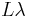$L\lambda$ spectral Radiance at the sensor's aperture, from equation... ToADD
•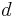$d$ - Earth-Sun distance in astronomical units, interpolated values
•$Esun$ - Mean solar exoatmospheric irradiance(s) (W/m2/μm), interpolated values
•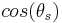$cos(\theta_s)$ - Solar_zenith_angle from the image acquisition's metadata

### Importing data

#### Importing

• Location creation based on georeferenced data

Once inside a Location that is defined by the spatial reference system in which the bands of interest are projected, they can be imported with the r.in.gdal module.

For example, GeoTIFF files can be imported by looping r.in.gdal over all of them

for TIF in echo *.tif; do r.in.gdal in=${TIF} out=${TIF%%.*}; done


To keep the raw material untouched, we create another Mapset inside the same Location and copy over the bands giving, optionally at the same time, a new name for each.

### Deriving Physical Quantities

Conversions implemented in the GRASS module i.worldview.toar

ToCleanUp

Converting Digital Numbers to Radiance/Reflectance requires knowledge about the sensor's specific spectral band parameters. In the following listed parameters (see below), the Effective Bandwidths and the Band-Averaged Solar Spectral Irradiances are extracted from the document Radiometric Use of WorldView-2 Imagery, Technical Note (2010) by Todd Updike and Chris Comp. The Absolute Calibration Factors are extracted from a WorldView-2 product, specifically from an image metadata file (extension .IMD).

Note, however, as stated in the above document,

  The absolute radiometric calibration factor is dependent on the specific band,
as well as the TDI exposure level, line rate, pixel aggregation, and bit depth
of the product. Based on these parameters, the appropriate value is provided in
the .IMD file. For this reason, care should be taken not to mix absolute radiometric
calibration factors between products that might have different collection conditions.


Pan_CalCoef=0.05678345		;	Pan_Width=0.2846000		;	Pan_Esun=1580.8140
Coastal_CalCoef=0.009295654	;	Coastal_CalCoef=0.04730000	;	Coastal_Esun=1758.2229
Blue_CalCoef=0.01260825		;	Blue_Width=0.05430000		;	Blue_Esun=1974.2416
Green_CalCoef=0.009713071	;	Green_Width=0.06300000		;	Green_Esun=1856.4104
Yellow_CalCoef=0.005829815	;	Yellow_Width=0.03740000		;	Yellow_Esun=1738.4791
Red_CalCoef=0.01103623		;	Red_Width=0.05740000		;	Red_Esun=1559.4555
RedEdge_CalCoef=0.005188136	;	RedEdge_Width=0.03930000	;	Red_Edge_Esun=1342.0695
NIR1_CalCoef=0.01224380		;	NIR1_Width=0.09890000		;	NIR_Esun=1069.7302
NIR2_CalCoef=0.009042234	;	NIR2_Width=0.09960000		;	NIR2_Esun=861.2866


The following examples exemplify the conversion of raw Blue band digital numbers into Radiance and Reflectance.

Converting a Blue Band (Digital Numbers) in to Spectral at-sensor Radiance in the correct units to be further used for the conversion in to unitless Reflectance:

# set the region
g.region rast=Blue_DNs -p

# convert DNs to spectral Radiance values
r.mapcalc "Blue_Radiance = ( (10000 * WorldView_Blue_DNs) / (... * ...) )"


#### Planetary Reflectance

The equation to derive Reflectance values incorporates in addition:

• The mathematical constant Pi PI=3.14159265358.
• The Earth-Sun distance in astronomical units which depends on the acquisition's day of year (DOY -- also referred to as Julian day, Ordinal_date) and can be retrieved from the following spreadsheet <http://landsathandbook.gsfc.nasa.gov/excel_docs/d.xls>.
• The mean solar exoatmospheric irradiance in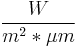$\frac{W}{m^2*\mu m}$. See 3rd column of (interplated) values given above.
• The cosine of the Solar Zenith Angle (SZA) at the time of the acquisition. The SZA can be calculated from its complementary Solar Elevation Angle (SEA) given in the image acquisition's metadata.

In the following example we accept as the acquisition's DOY=... and SEA=.... Hence, we get the Earth-Sun distance ESD=... and SZA = ... deg.

In any case, note that Top-of-Atmosphere Reflectance does not account for topographic, atmospheric, or BRDF differences.

Converting the in-Blue spectral band at-sensor Radiance in to Planerary Reflectance:

PI=...; ESD=...; BAND_Esun=...; SZA=...
r.mapcalc "Blue_Reflectance = ( ${PI} * Blue_Radiance *${ESD}^2 ) / ( ${BAND_Esun} * cos(${SZA}) )"


#### Automatising Conversions

The conversion process can be scripted to avoid repeating the same steps for each band separately.

##### Python

A custom python script, performing the operations of interest, might be like i.worldview.toar (for GRASS 7.x)

##### Bash

In bash, such a script might be as the following example. Note, however, in this example script constants, band parameters and acquisition related metadata are hard-coded!

#!/bin/bash

# π, first 11 decimals
PI=3.14159265358

# Acquisition's Day of Year and estimated Earth-Sun Distance
DOY=100; ESD=1.00184

# Sun Zenith Angle based on the acquisition's Sun Elevation Angle
SEA=53.8; SZA=$(echo "90 -${SEA}" | bc )

# some echo
echo "Acquisition-specific parameters"
echo "Day of Year: ${DOY}, Earth-Sun Distance:${ESD}, Sun Zenith Angle: ${SZA}" echo -e "\n" # 1st column: Absolute Calibration Factors # 2nd column: Spectral Band Effective Bandwidth, Δλ # 3rd column: Band-Averaged Solar Spectral Irradiance [W/sq.m./micro-m] K_Pan=0.05678345 ; Pan_Width=0.2846000 ; Pan_Esun=1580.8140 K_Coastal=0.009295654 ; Coastal_Width=0.04730000 ; Coastal_Esun=1758.2229 K_Blue=0.01260825 ; Blue_Width=0.05430000 ; Blue_Esun=1974.2416 K_Green=0.009713071 ; Green_Width=0.06300000 ; Green_Esun=1856.4104 K_Yellow=0.005829815 ; Yellow_Width=0.03740000 ; Yellow_Esun=1738.4791 K_Red=0.01103623 ; Red_Width=0.05740000 ; Red_Esun=1559.4555 K_RedEdge=0.005188136 ; RedEdge_Width=0.03930000 ; RedEdge_Esun=1342.0695 K_NIR1=0.01224380 ; NIR1_Width=0.09890000 ; NIR1_Esun=1069.7302 K_NIR2=0.009042234 ; NIR2_Width=0.09960000 ; NIR2_Esun=861.2866 # Bands Spectral_Bands="Pan Coastal Blue Green Yellow Red RedEdge NIR1 NIR2" # loop over all bands for BAND in${Spectral_Bands}; do

# some echo
echo -e "Processing the \"${BAND}\" spectral band" # set band parameters as variables eval BAND_Width="${BAND}_Width"
echo "* Band-specific radiometric parameters set to K=${!K_BAND}, Bandwidth=${!BAND_Width}"

# set region
g.region rast=${BAND}_DNs # conversion to Radiance based on (1) -- attention: 32-bit calculations required echo "* Converting${BAND} Digital Numebrs to Radiance..." | tr -d "\n"
r.mapcalc "${BAND}_Radiance = ( double(${!K_BAND}) * ${BAND}_DNs ) /${!BAND_Width}"

# report range
echo "* Reporting range of the ${BAND} spectral radiance: " | tr -d "\n" # r.info -r "${BAND}_Radiance"
r.info -r "${BAND}_Radiance" | tr "\n" "," | cut -d"," -f1,2 | sed 's/,/,\ /' # add info r.support map=${BAND}_Radiance \
title="" \
units="W / sq.m. / μm / ster" \
description="Top-of-Atmosphere echo ${BAND} band spectral Radiance [W/m^2/sr/μm]" \ source1='"Radiometric Use of WorldView-2 Imagery, Technical Note (2010)", by Todd Updike & Chris Comp' # Esun eval BAND_Esun="${BAND}_Esun"

# some echo
echo "* Parameters required for the conversion: Esun= ${!BAND_Esun}, ESD=${ESD}, ${SZA}" # calculate ToAR echo "* Converting${BAND}_Radiance to Top of Atmosphere Reflectance..." | tr -d "\n"
r.mapcalc "${BAND}_ToAR = \ (${PI} * ${BAND}_Radiance *${ESD}^2 ) / ( ${!BAND_Esun} * cos(${SZA}) )"

# report range
echo "* Reporting range of the ${BAND} spectral radiance: " | tr -d "\n" r.info -r${BAND}_ToAR | tr "\n" "," | cut -d"," -f1,2 | sed 's/,/,\ /'

r.support map=${BAND}_ToAR \ title="echo${BAND} band (Top of Atmosphere Reflectance)" \
units="Unitless planetary reflectance" \
description="Top of Atmosphere echo ${BAND} band spectral Reflectance (unitless)" \ source1='"Radiometric Use of WorldView-2 Imagery, Technical Note (2010)", by Todd Updike & Chris Comp, Digital Globe' \ source2="Digital Globe" \ history="PI=3.14159265358; K=${!K_BAND}; Bandwidth=${!BAND_Width}; ESD=${ESD}; Esun=${!BAND_Esun}; SZA=${SZA}"

echo -e "\n"

done


## Post-Processing

Having beforehand satellite image data expressed in physical quantities (radiance or reflectance) is preferred to follow-up with digital image analysis techniques. A few common post-processing practices are Contrast-Enhancement, Pan-Sharpening and creating Pseudo- or True-Color Composites. Other well known enhancing manipualtions to support the analyses of satellite imagery, include deriving Vegetation Indices, transforming multi-spectral data based on PCA and Segmenting images.

### Pan-Sharpening

Pan-Sharpening / Fusion is the process of merging high-resolution panchromatic and lower resolution multi-spectral imagery. GRASS 7 holds a dedicated pan-sharpening module, i.pansharpen which features three techniques for sharpening, namely the Brovey transformation, the classical IHS method and one that is based on Principal Components Analysis (PCA). Another algorithm deriving excellent detail and a realistic representation of original multispectral scene colors, is the High-Pass Filter Addition (HPFA) technique. It is implemented via the i.fusion.hpf (src) add-on (for GRASS 6, please refer to a bash shell script https://github.com/NikosAlexandris/i.fusion.hpf.sh which is, however, unmaintained).

One approach inside GRASS-GIS to get an acceptable color-balanced composite image after Pan-sharpening 11-bit IKONOS spectral bands comprises the following steps

1. rescale the 11-bit WorldView spectral bands to 8-bit ranging in [0, 255] (r.rescale)
2. pan-sharpen with any of the featured methods (Brovey, IHS, PCA) (i.pansharpen)
3. color-balance by using the i.landsat.rgb module or manually adjusting the color tables of the bands of interest
4. create a composite image by using the r.compose module

#### Automatising Sharpening & RGB Composition

An experimental bash script to automatise the sharpening/fusion and RGB composition process is demonstrated here. The script uses the Spectral Reflectance values (double precision values), gained from previous steps (see above), and produces Pan-sharpened images by applying all of the three methods that i.pansharpen offers. In addition, it attempts to produce True-Color composite images, without and with re-balancing the color tables by using the i.landsat.rgb module.

Initial tests indicate that at least one of the three sharpening methods, combined with re-balancing, produces very clear and balanced True-Color composites. Note, there is no 100% confidence that it will produce nice looking True-Color composites. Some of the methods might simply work, others might deliver fancy-colored images. As usual, some manual action might be required to get the re-balancing to work as best as possible.

The script can be expanded in terms of using more inputs by utilising bash's positional parameters. However, care has to be taken to alter the instruction that concerns the conversion from double precision values to 8-bit integers.

...